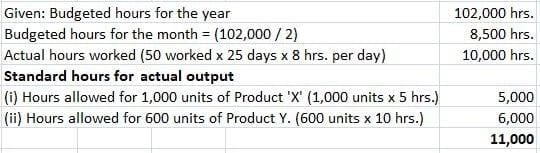## What Are Control Ratios?

Under a budgetary control system, actual performance is compared to budgeted performance to enable the identification of variances.

Deviations or variances may be favorable or unfavorable, and they may be expressed in terms of absolute figures or in terms of ratios.

When deviations or variances are expressed in terms of ratios, these ratios are referred to as control ratios.

## Types of Control Ratios

The types of control ratios are as follows:

1. Capacity ratio
2. Activity ratio
3. Efficiency ratio

## Capacity Ratio

The capacity ratio is also known as the actual usage of budgeted capacity ratio. It shows the relationship between the actual number of working hours and the budgeted number of working hours.

This ratio indicates the extent to which available facilities have actually been utilized during the budget period.

### Formula

Capacity ratio = (Actual hours / Budgeted hours) x 100

## Activity Ratio

The activity ratio is the number of standard hours equivalent to the work produced expressed as a percentage of the budgeted standard hours. This ratio measures the level of activity at which a business is operating.

### Formula

Activity ratio = (Actual production in standard hours / Budgeted production in standard hours) x 100

## Efficiency Ratio

The efficiency ratio is the number of standard hours equivalent to the work produced expressed as a percentage of the actual hours spent in production. This ratio measures the efficiency of a firm’s operations.

### Formula

Efficiency ratio = (Actual production in standard hours / Actual hours worked) x 100

## Interpretation of Control Ratios

The results of control ratios may be interpreted as follows:

 Results Interpretation 100% No deviation Above 100% Favorable deviation Below 100% Unfavorable deviation

## Example

Product X takes 5 hours to make and Product Y requires 10 hours.

In a month of 25 effective days of 8 hours a day, 1,000 units of X and 600 units of Y were produced. The company employs 50 workers in the production department, and the budgeted hours for the year are 102,000.

Required: Calculate the following control ratios:

• (A) Capacity ratio
• (B) Activity ratio
• (C) Efficiency ratio

### Solution(A) Capacity ratio = (Actual hours / Budgeted hours) x 100
= (10,000 hrs. / 8,500 hrs.) x 100
= 117.65%
(B) Activity ratio = (Actual production in standard hours / Budgeted production in standard hours) x 100
= (11,000 hrs. / 8,500 hrs.) x 100
= 129.41%
(C) Efficiency ratio = (Actual production in standard hours / Actual hours worked) x 100
= (11,000 hrs. / 10,000 hrs.) x 100
= 110%

### What are control ratios?

Under a budgetary control system, actual performance is compared to budgeted performance to enable the identification of variances. When deviations or variances are expressed in terms of ratios, these ratios are referred to as control ratios.

### What are the types of control ratios?

The types of control ratios are as follows: 1. Capacity ratio 2. Activity ratio 3. Efficiency ratio

### What is a capacity ratio?

The capacity ratio is also known as the actual usage of budgeted capacity ratio. It shows the relationship between the actual number of working hours and the budgeted number of working hours.

### What is an activity ratio?

The activity ratio is the number of standard hours equivalent to the work produced expressed as a percentage of the budgeted standard hours. This ratio measures the level of activity at which a business is operating.

### What is an efficiency ratio?

The efficiency ratio is the number of standard hours equivalent to the work produced expressed as a percentage of the actual hours spent in production. This ratio measures the efficiency of a firm’s operations.

True is a Certified Educator in Personal Finance (CEPF®), a member of the Society for Advancing Business Editing and Writing, contributes to his financial education site, Finance Strategists, and has spoken to various financial communities such as the CFA Institute, as well as university students like his Alma mater, Biola University, where he received a bachelor of science in business and data analytics.

To learn more about True, visit his personal website, view his author profile on Amazon, his interview on CBS, or check out his speaker profile on the CFA Institute website.